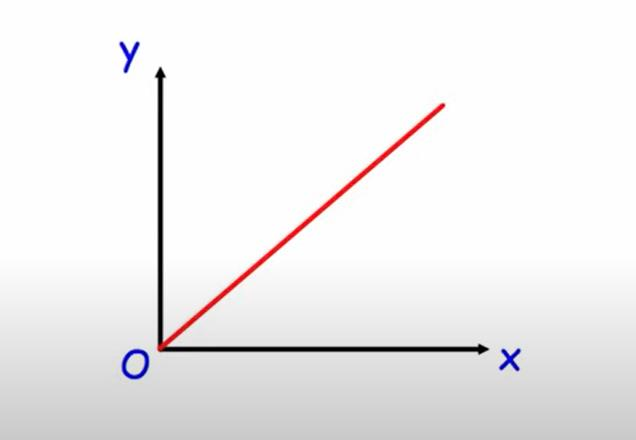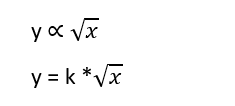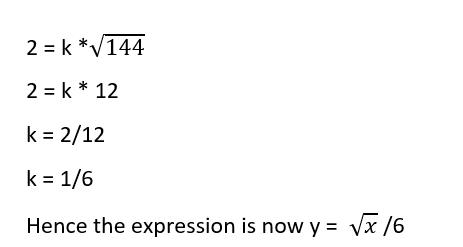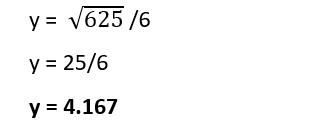Education Talk Stuff

# An Introduction To Proportion: Its Definition, Types, And ExamplesProportion is determined by fractions. The fraction is expressed as a/b, the ratio as a: b, and the proportion as the equality of two ratios. Proportions are a crucial subject in mathematics as well as other social sciences.

In real life, the notion of proportions is applied in business when dealing with money transactions, and at home when we make certain dishes.

## What is meant by proportion?

Proportion is defined as the equality between two ratios. It is written as

a: b :: c: d

or

a: b = c: d

where a and d are called extremes and b and c are called means.

Rule:

the Product of means = the product of extremes

## Types of proportion

The two main types of proportions are

• Direct Proportion
• Inverse Proportion

### 1.     Direct Proportion

In direct proportion, the relationship is built between the quantities in such a way with the increase of one quantity case increases the other qualities. Similarly when one quantity is decreased then the other qualities also decrease.

If y is directly proportional to z then it is represented as

y ∝ z

The relationship between food and people, hard work, and marks are related directly to each other.

Example:

If the cost of one dozen of eggs is \$84. Find the cost of eggs if we want to purchase 15 eggs.

Solution:

Method 1:

Step 1: Write the given data according to the information.

Price: Eggs

84: 12

x: 15

Step 2: The relationship between cost and quantity is a direct proportion.

x/84 = 15/ 12

x = (15/12) *84

x = \$105

The cost of the 15 eggs is \$105.

Method 2: Unit method

Step 1: First find the cost of one egg.

The cost of 12 eggs = \$ 84

Cost of 1 egg = \$ 84/12

Cost of 1 egg = \$ 7

Step 2: For the cost of 15 eggs we just multiplied by the cost of one egg.

So the cost of 15 eggs = 15 * 7

Cost of 15 eggs = \$ 10

### 2.     Inverse Proportion

The Inverse proportion is defined as the increase in one quantity that will cause a decrease in the other quantity. Similarly, a decrease in one quantity will cause an increase in the other quantity.

If x is inversely proportional to y. It is represented by

x ∝ 1/y

The relationship between workers and days, expenditures, and savings are some examples of inverse relations.

Example:

If there are 20 people working together on a project which will be completed in 10 days. Find the number of days if the number of workers is half.

Solution:

Step 1: Write the given data according to the information

Number of workers: Number of days

20   :  10

10   :  x

Step 2: the relationship between cost and quantity is a direct proportion.

x/10 = 20/ 10

x = (20/10) *10

x = 20 days

The total number of days is double if the workers are half.Example 1:

y is directly proportional to x. The value of y = 100 and x = 10. If the value of x = 100 then finds the value of y.

Solution:

Step 1: Write the given statement in the proportional form

y ∝ x

y = k * x

Where k is known as the constant of proportionality.

Step 2: Put the value of x and y to find the value of constant k.

y = k * x

100 = k * 10

100/10 = k

10 = k

Step 3: Now put the value of k and x to evaluate the value of y.

y = 10 * 100

y = 1000

The graph represented the relationship y ∝ x.Example 2:

The y deviates inversely to the x. The value of x = 7 and y = 84. Show the relation by graph and find the value of x if y = 100.

Solution:

Step 1: Write in the form of inverse proportion notation

y ∝ 1/x

y = k * 1/x

Where k is known as the constant of proportionality.

Step 2:  Find the value of constant k.

84 = k/7

84 * 7 =k

588 = k

Step 3: For the value of x when y = 100.

100 = 588/x

x*100 = 588

x = 588/100

x = 5.88

The graph represents the proportion y 1/x.The concept of proportion is very easy to understand. A proportion calculator is a helpful tool to solve the proportion problems.

Example 3:

If y is directly proportional to the square root of x.

• find the expression between them
• find the value of the constant of proportionality if x= 144 and y = 2
• find the value of y if x = 625

Solution:

Step 1: Write the given statement in a mathematical wayThis is the relation between y and x.

Step 2: Now for the value of k but the given values of x and y in the relation.Step 3: Now put the value of x to find the value of y.The graph representation of the relation y ∝ √x## Application in real life

The proportion is used widely in our daily life. Some of the common uses are described below.

 ·         Cycling Speed and Strength ·         Speed of the fan ·         Balloon Inflation ·         Toys with Keys ·         Recipe Ingredients and Number of Customers ·         The Melting Rate of Ice Cream and Temperature ·         Fruit Weight and Cost ·         Number of goals and points scored ·         Farming and land are also available. ·         Flame and Temperature ·         Time and distance traveled by a vehicle ·         A person’s age and height ·         Object Height and Shadow ·         Petrol Consumption and Travel Distance
 ·         Food Requirements in a Hostel ·         Earnings of a Worker per Day ·         Cost of an Object vs. the Number of Objects Purchased

## Summary

In this post, we have learned about proportion, examples with graphs, and different methods to solve problems more easily. Now you can understand the problems and be able to find the proper solution.

 Rate This Post